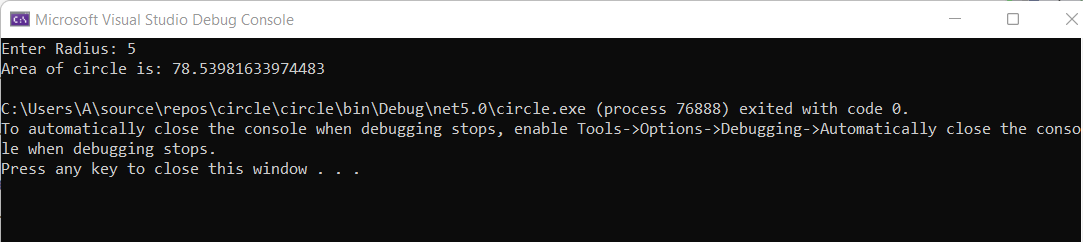# How to Calculate Area of Circle in C#?

• Last Updated : 22 Jun, 2022

C# is a general-purpose, modern and object-oriented programming language pronounced as “C Sharp”. In this article, we will see how to calculate the area of a circle in C#.

The area of a circle can be evaluated using the formula:

Area of circle  = pi * r * r   //where r is the radius of circle

Example 1:

## C#

 `// Program to implement area of circle in C#``using` `System;``class` `Circle``{``    ``static` `void` `Main(``string``[] args)``    ``{``        ``Console.Write(``"Enter Radius: "``);``        ``double` `rad = Convert.ToDouble(Console.ReadLine());``        ``double` `area = Math.PI * rad * rad;``        ``Console.WriteLine(``"Area of circle is: "` `+ area);``    ``}``}`

Output:### Code Explanation:

The formula used to calculate the circle area: π * r * r. Where, r is the radius and π is the mathematical constant Pi. We will take the value of radius from the user as input. For π, we can either define a variable and assign it up to a specific decimal place or we can use Math.PI constant that is available in C#.

• The Radius is a double value to keep the radius of the circle.
• The area is double to save the area of the circle.
• The final area is stored in the double area.
• Finally, we are writing down the area of a circle.
My Personal Notes arrow_drop_up